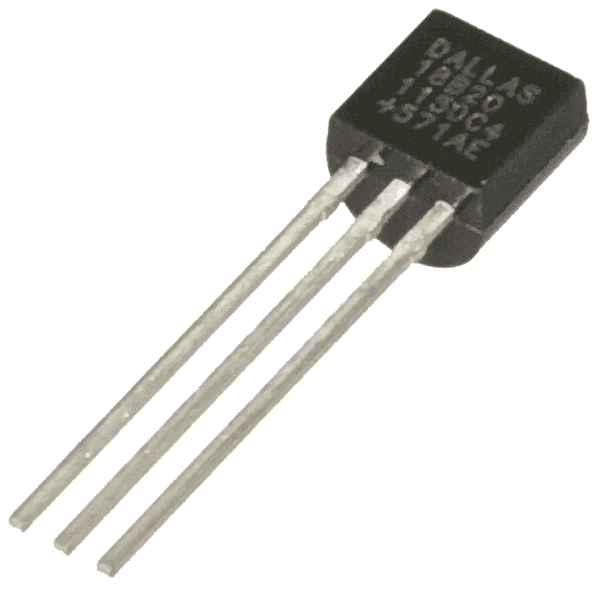## Sunday, 24 November 2013

### Interfacing a BMP085 Digital Pressure sensor to the Raspberry Pi

I recently bought a sensor with a BMP085 Digital Pressure sensor on it so I thought I'd write a post on how to read the data from the Raspberry Pi in Python over I2C.

### Python code

Below is simple test code to initialise the sensor and then continuously loop around reading the temperature and air pressure.
``````#!/usr/bin/python
import smbus
import time

bus = smbus.SMBus(0) # or bus = smbus.SMBus(1) if you have a revision 2 board

val = (high << 8) + low
return val

if (val >= 0x8000):
return -((0xffff - val) + 1)
else:
return val

def twos_compliment(val):
if (val >= 0x8000):
return -((0xffff - val) + 1)
else:
return val

def get_word(array, index, twos):
val = (array[index] << 8) + array[index+1]
if twos:
return twos_compliment(val)
else:
return val

def calculate():
# This code is a direct translation from the datasheet
# and should be optimised for real world use

#Calculate temperature
x1 = ((temp_raw - ac6) * ac5) / 32768
x2 = (mc * 2048) / (x1 + md)
b5 = x1 + x2
t = (b5 + 8) / 16

# Now calculate the pressure
b6 = b5 - 4000
x1 = (b2 * (b6 * b6 >> 12)) >> 11
x2 = ac2 * b6 >> 11
x3 = x1 + x2
b3 = (((ac1 * 4 + x3) << oss) + 2) >> 2

x1 = (ac3 * b6) >> 13
x2 = (b1 * (b6 * b6 >> 12)) >> 16
x3 = ((x1 + x2) + 2) >> 2
b4 = ac4 * (x3 + 32768) >> 15
b7 = (pressure_raw - b3) * (50000 >> oss)
if (b7 < 0x80000000):
p = (b7 * 2) /b4
else:
p = (b7 / b4) *2
x1 = (p >> 8) * (p >> 8)
x1 = (x1 * 3038) >> 16
x2 = (-7357 * p) >> 16
p = p + ((x1 + x2 + 3791) >> 4)
return(t,p)

oss = 3              # Ultra high resolution
temp_wait_period = 0.004
pressure_wait_period = 0.0255 # Conversion time

# The sensor has a block of factory set calibration values we need to read
# these are then used in a length calculation to get the temperature and pressure
ac1 = get_word(calibration, 0, True)
ac2 = get_word(calibration, 2, True)
ac3 = get_word(calibration, 4, True)
ac4 = get_word(calibration, 6, False)
ac5 = get_word(calibration, 8, False)
ac6 = get_word(calibration, 10, False)
b1 =  get_word(calibration, 12, True)
b2 =  get_word(calibration, 14, True)
mb =  get_word(calibration, 16, True)
mc =  get_word(calibration, 18, True)
md =  get_word(calibration, 20, True)

while True:
write_byte(0xF4, 0x2E)          # Tell the sensor to take a temperature reading
time.sleep(temp_wait_period)    # Wait for the conversion to take place

write_byte(0xF4, 0x34 + (oss << 6)) # Tell the sensor to take a pressure reading
time.sleep(pressure_wait_period)    # Wait for the conversion to take place
pressure_raw = ((read_byte(0xF6) << 16) \

temperature, pressure = calculate()
print time.time(), temperature / 10., pressure / 100.
time.sleep(1)
``````
To get a reading out of the sensor you first have to read the factory set calibration block (lines 080-090).  This is different for each device and is used in the lengthy calculations for both temperature and pressure.  The function calculate() is just a direct translation of the code presented in the datasheet, I don't understand what it's doing but it gives us the required values.

### Testing the sensor and the code

To test everything was working OK I saved the above code to a file called read-pressure.py, ran it and re-directed the output to a file
`sudo ./read-pressure.py > pressure-test.dat`
I then slowly walked up and down the stairs in my house to get some data.  Then plotted the data with the following gnuplot program

```  set terminal wxt persist size 800,800 background '#000000'
set style line 99 linecolor rgb "#ffffff" linetype 0 linewidth 2
set key top right textcolor linestyle 99
set grid linestyle 99
set border linestyle 99

set yrange  [16.4:17.2]
set y2range [1003.5:1005]
set y2tics

plot filename using 1:2 axes x1y1 title "True temp" w l ,\
filename using 1:3 axes x1y2 title "True pressure" w l, \
filename using 1:3 axes x1y2 title "Smoothed" smooth bezier
```

Here is the command to generate the plot below
`gnuplot -e "filename='pressure-test.dat'" gnuplot-pressure.plg`
You can see the pressure dropping as I went up the stairs and then back down again.  You can see the temperature went up slightly too which I think was just heat from my hand slowly raising it.Sensor response as I walked up and down the stairs
To calculate altitude (height above ground) I used the first (p0) and the lowest (p) readings from the output and plugged them into the following formula, again this is taken from the datasheet.

This gave me a height of 2.86m, I was surprised to get a significant reading by just walking up and down the stairs so when I finally add it to a quad-copter I should get good results.

#### 1 comment:

1.Hello. Do you convert the script in python 3 ?
With python3 we have many error like this unsupported operand type(s) for >>: 'float' and 'int'

### Temperature logging with a DS18B20 and a Raspberry Pi

I wanted to do some temperature logging so I hooked up a DS18B20 temperature sensor to a Raspberry Pi. About the DS18B20 Dallas DS18B...